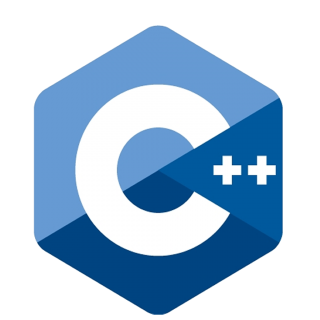15 Nov 2023
69 ViewsLearn via Video Course & by Doing Hands-on Labs

## C++ Function Overriding: An Overview

We have seen what is `function overloading` in detail in the C++ function overloading tutorial. We have also understood `C++``function overriding` in brief in the previous tutorial, polymorphism in C++. In this C++ tutorial, we will go a little deeper and analyze `function overriding` from various aspects. For practical understanding and hands-on, refer to our C++ Certification course.

## What is Function Overriding in C++?

As seen in the inheritance in C++ tutorial, the derived classes inherit the features i.e. attributes and methods of the parent class. When you redefine any base class method with the same signature i.e. same return type, number, and type of parameters in the derived class, it is known as `function overriding` in C++.

The function call by the object of the derived class invokes the derived class method instead of the base class. Thus, the derived class method overrides the base class method. Hence,

• The function in the derived class becomes the overriding function
• The function in the base class becomes the overridden function

From here onwards, we will use the terms`overriding` and `overridden` method to refer to the same methods of derived class and base class respectively.

#### Syntax

``````class Parent{

access_modifier:

// overridden function
return_type name_of_the_function(){}

};

}

class child : public Parent {

access_modifier:

// overriding function
return_type name_of_the_function(){}

};

}
``````

#### Example

``````
#include <iostream>
using namespace std;

class DotNetTricks {
public:
void print() { //overridden function
cout << "Welcome to DotNetTricks" << endl;
}
};

class ScholarHat : public DotNetTricks {
public:
void print() { //overriding function
cout << "Welcome to ScholarHat" << endl;
}
};

int main() {
ScholarHat obj1;

// Call the print() function of the ScholarHat class
obj1.print();

return 0;
}
``````

#### Output

``````Welcome to ScholarHat
``````

We have already seen this illustration in the previous tutorial while studying the runtime polymorphism in C++. Here, what if we call the `print()` function from an object of the `DotNetTricks` class? If we do so, the `print()` function will not get overridden because the object of the base class will always invoke the method of the base class and not the derived class.

Make sure that you are thorough with the concepts of pointers in C++, functions in C++, Call by Value and Call by Reference in C++, and the OOPs concepts in C++. If not; go back, refer them, and then come here.

## Variations in C++ Function Overriding

### Call Overridden Function From Derived Class

#### Example

``````
#include <iostream>
using namespace std;

class DotNetTricks {
public:
void print() { // overridden function
cout << "Welcome to DotNetTricks" << endl;
}
};

class ScholarHat : public DotNetTricks {
public:
void print() { // overriding function
cout << "Welcome to ScholarHat" << endl;
DotNetTricks::print(); // call overridden function
}
};

int main() {
ScholarHat obj1;
obj1.print();

return 0;
}
``````

In this program, we have called the overridden function inside the derived class, `ScholarHat` itself. When you call `obj1.print()`, it first executes the overridden `print()` method in the `ScholarHat` class and then explicitly calls the overridden function from the base class using `DotNetTricks::print()`. Thus, we can use the scope resolution operator `::` to access the overridden function of the base class.

#### Output

``````Welcome to ScholarHat
Welcome to DotNetTricks
``````

### Access Overridden Function to the Base Class

#### Example

``````
#include <iostream>
using namespace std;

class DotNetTricks {
public:
void print() { // overridden function
cout << "Welcome to DotNetTricks" << endl;
}
};

class ScholarHat : public DotNetTricks {
public:
void print() { // overriding function
cout << "Welcome to ScholarHat" << endl;
}
};

int main() {
ScholarHat obj1, obj2;
obj1.print();

// Access the print() function of the Base class
obj2.DotNetTricks::print();

return 0;
}
``````

In the above C++ code, we have created two `ScholarHat` objects, `obj1` and `obj2`. `obj1.print()` calls the overridden `print()` function in the `ScholarHat` class, while `obj2.DotNetTricks::print()` explicitly accesses and calls the `print()` function of the `DotNetTricks` base class in the `main()` function.

#### Output

``````Welcome to ScholarHat
Welcome to DotNetTricks
``````

### Call Overridden Function Using Pointer

#### Example

``````
#include <iostream>
using namespace std;

class DotNetTricks {
public:
void print() { //overridden function
cout << "Welcome to DotNetTricks" << endl;
}
};

class ScholarHat : public DotNetTricks {
public:
void print() { // overriding function
cout << "Welcome to ScholarHat" << endl;
}
};

int main() {
ScholarHat sobj1;

// Pointer of the DotNetTricks type that points to sobj1
DotNetTricks* ptr = &sobj1;

// Calls the function of DotNetTricks using ptr
ptr->print();

return 0;
}
``````
• In the above code, a pointer of `DotNetTricks` type named `ptr` points to the `ScholarHat` object `sobj1`
• When we call the `print()` function using `ptr`, it calls the overridden function and not the overriding function.
• The reason is even though `ptr` points to a `ScholarHat` object, it is actually of `DotNetTricks` type. So, it calls the member function of the `DotNetTricks` class.
• Thus, the `print()` function of the derived class, `ScholarHat` is not overriding the `print()` of the base class, `DotNetTricks`
• Here comes the need for `virtual functions` in the base class which we will see in the next tutorial, C++ Virtual Functions.

#### Output

``````Welcome to DotNetTricks
``````

With this, we have completed two important techniques of polymorphism, `function overloading` and `function overriding` in C++. Now we have completed almost half of the OOP concepts. There are a lot of things to understand in theOOPs concepts. Therefore get thorough with your concepts as much as you can. To sharpen your skills, enrol in our C++ Certification.